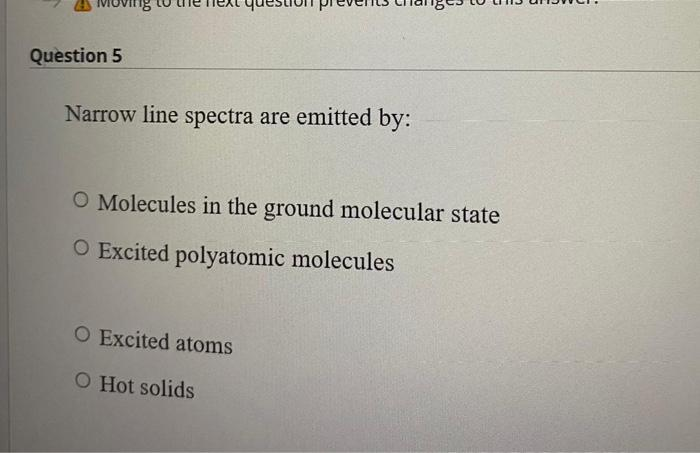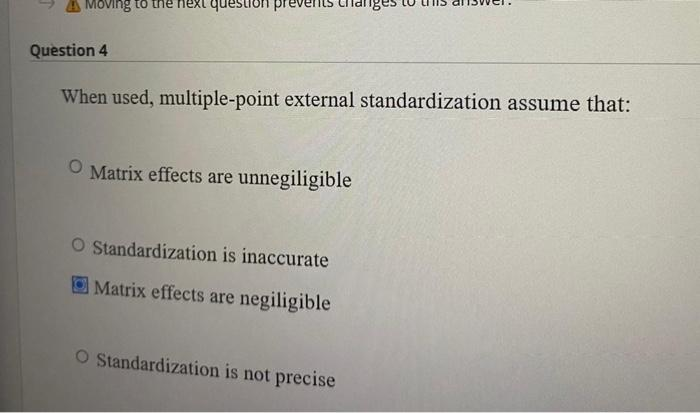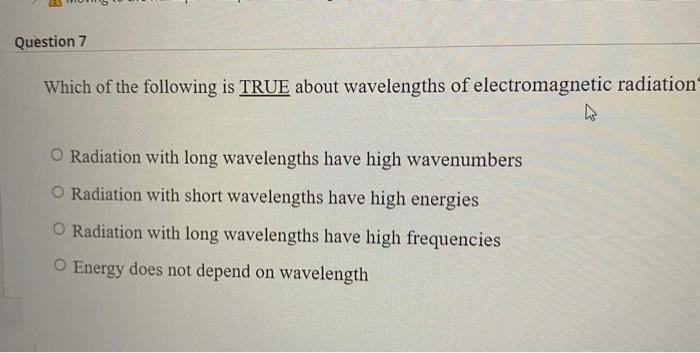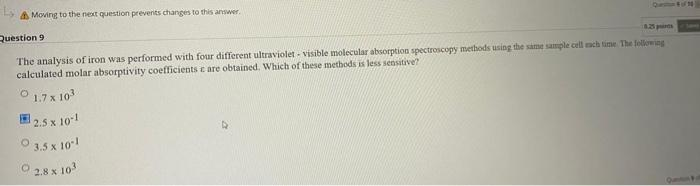Home / Expert Answers / Chemistry / solve-it-narrow-line-spectra-are-emitted-by-molecules-in-the-ground-molecular-state-excited-polyato-pa211

# (Solved): solve it Narrow line spectra are emitted by: Molecules in the ground molecular state Excited polyato ...

solve itNarrow line spectra are emitted by: Molecules in the ground molecular state Excited polyatomic molecules Excited atoms Hot solids When used, multiple-point external standardization assume that: Matrix effects are unnegiligible Standardization is inaccurate Matrix effects are negiligible Standardization is not precise Which of the following is TRUE about wavelengths of electromagnetic radiation Radiation with long wavelengths have high wavenumbers Radiation with short wavelengths have high energies Radiation with long wavelengths have high frequencies Energy does not depend on wavelength uestion 9 The analysis of iron was performed with four different ultraviolet - visible molecular absorption spectroscogy methods using ithe sime sainele cell meh time The folkinial calculated molar absorptivity coefficients $$x$$ are obtained. Which of these metbods is less sensitive? $$1.7 \times 10^{3}$$ $$2.5 \times 10^{-1}$$ $$3.5 \times 10^{-1}$$ $$2.8 \times 10^{3}$$

We have an Answer from Expert